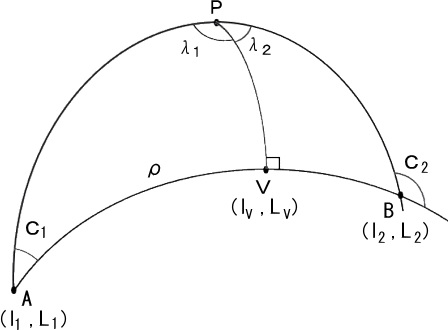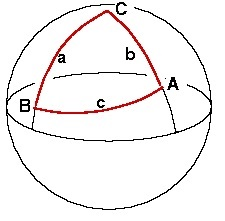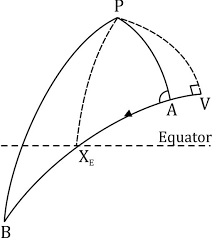# What is meaning of Great Circle Sailing ?Written by

Great circle sailing involves the solution of courses, distances, and points along a great circle between two points.

Great Circle Sailing is used for long ocean passages. For this purpose, the earth is considered a perfect spherical shape; therefore, the shortest distance between two points on its surface is the arc of the great circle containing two points. As the track is the circle, so the course is constantly changing, and the track must be broken down into a series of short rhumb lines at frequent intervals that can be used to sail on the Mercator chart. In doing this, the navigator would use the Gnomonic charts combined with the Mercator charts to draw the sailing track.###### In Other words, we can understand the great circle as:

A great circle is the shortest path between two points along the surface of a sphere. A great circle is the intersection of the surface with a plane passing through the center of the planet.

The equator and all meridians are great circles. All great circles other than these do not have a constant azimuth, the spherical analog of slope; they cross successive meridians at different angles. The Gnomonic Projection represents arbitrary great circles as straight lines.

Great circles are examples of geodesics. A geodesic is the shortest possible path constrained to lie on a curved surface, independent of the choice of a coordinate system.###### Find Dist:

COS AB = (COS PA X COS PB) + (SIN PA X SIN PB X COS P)

###### Find Initial Course:

( COS PB – COS AB X COS PA)
COS A = ———————————–
(  SIN AB X SIN PA)

###### Find Final Course:

( COS PA – COS AB) X ( COS PB)
COS B = ————————————–
(SIN AB X SIN PB)The initial course always has same name as the initial latitude and east or west direction of the course. The final course always has the opposite name from final latitude unless initial position and final position are in different hemispheres, when the final will have same name as final latitude and east or west direction of the course.